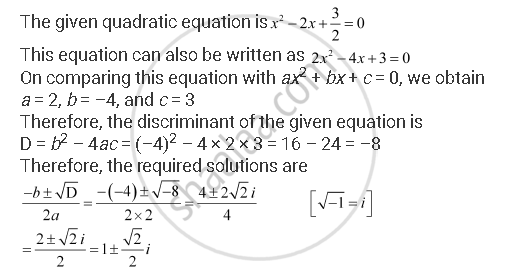CBSE (Arts) Class 11CBSE
Share

# Solve the Quadratic Equationequation Xsquare2 -2x + 3/2 = 0 - CBSE (Arts) Class 11 - Mathematics

#### Question

Solve the equation   x^2 -2x + 3/2 = 0

#### SolutionIs there an error in this question or solution?

#### Video TutorialsVIEW ALL 

Solution Solve the Quadratic Equationequation Xsquare2 -2x + 3/2 = 0 Concept: Quadratic Equations.
S